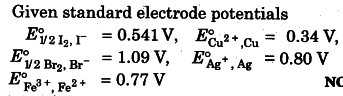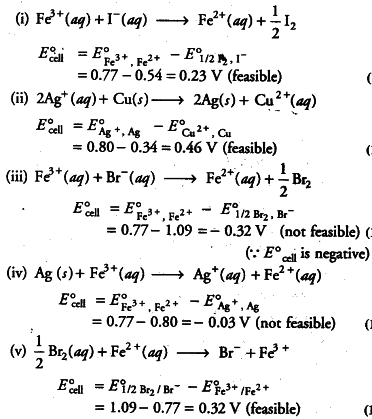# Using the standard electrode potentials

Using the standard electrode potentials, predict if the reaction between the following is feasible.
(i) {{Fe}^{3+}}(aq) and {{I}^{-}}(aq) (ii) {{Ag}^{+}}(aq) and Cu (s) (iii) {{Fe}^{3+}}(aq) and {{Br}^{-}}(aq) (iv) Ag(s) and {{Fe}^{3+}}(aq)
(v) Br _{ 2 }(aq) and {{Fe}^{2+}}(aq)The reaction will be feasible, if emf of the cell reaction is positive.